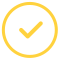effortlessly.

# What Is A Normal Line Calculator?

Calculus calculations don't have to get you all worked up every day. With this equation of normal line calculator, you can calculate your derivatives with ease and attain top grades in your math homework.

A normal line in calculus refers to a line along a normal vector perpendicular to some tangent line. Take a case where we have a tangent line to a function. The tangent line and function intersect at the point of tangency. The normal line is that which passes through that same point that is perpendicular to the tangent line.

When two lines are perpendicular, their slopes form negative reciprocals. With the normal line equation calculator, this work is simplified for you. All you have to do is key in the required values of the function, and you are good to go!

On top of that, the equation of the normal line calculator gives you the expected graph. You are thus assured of accuracy when submitting your final math paper.

## How To Find The Equation of The Normal Line

It's pretty easy to find equation of line normal calculator. The rule for perpendicular lines applies when using the slope of normal line calculator. Note that normal lines are perpendicular to the tangent line when the normal intersects with the curve.

Therefore, the slope of each normal line and corresponding tangent are opposite to each other. You will find this with a derivative.

The line's equation, normal to the curve, is derived as follows:

Since the slope's tangent line is m=f′(x), then the slope of the normal line is m=−1/f′(x)

### Equation Of Normal Line at Point Calculator – Examples

1. Find the normal line equation to the graph of f(x) = at the point (−1, 2).

Solution

y - y1 = m(x – x1)

y – 2 = 2 (x+1)

y – 2 = 2x + 2

2x - y = -4

2. Calculate the equation of the tangent line to the graph of f(x) = √(x2+3) at the point (−1, 2).

Solution

y - y1 = m(x – x1)

y – 2 = -1/2 (x+1)

2y – 4 = -x -1

x + 2y = 3

### How To Use The Normal Line Graph Calculator

The normal line to a curve calculator simplifies these tedious calculations above and enables you to arrive at your answer in a fraction of a second. You can solve even the most complex normal line graph equation with the simple steps below:

• First, enter the function in the specified field
• Enter the point of intersection
• Hit the 'CALCULATE' buttonAwesome. Now you'll never miss out.